SNOSA87C October   2003  – October 2016

PRODUCTION DATA.

1. Features
2. Applications
3. Description
4. Revision History
5. Pin Configuration and Functions
6. Specifications
7. Detailed Description
8. Application and Implementation
1. 8.1 Application Information
2. 8.2 Typical Application: 2.7-V Single Supply 2:1 MUX
9. Power Supply Recommendations
10. 10Layout
11. 11Device and Documentation Support
12. 12Mechanical, Packaging, and Orderable Information

• DBV|5

## 6 Specifications

### 6.1 Absolute Maximum Ratings

over operating free-air temperature range (unless otherwise noted)(1)(2)
MIN MAX UNIT
Supply voltage (V+ - V) 12.6 V
Voltage at INPUT and OUTPUT pins V −0.8 V+ + 0.8 V
Output short-circuit duration See(3), (4)
Junction temperature(5) 150 °C
Soldering information Infrared or convection (20 seconds) 235 °C
Wave soldering lead temperature (10 seconds) 260 °C
Storage temperature, Tstg –65 150 °C
Stresses beyond those listed under Absolute Maximum Ratings may cause permanent damage to the device. These are stress ratings only, which do not imply functional operation of the device at these or any other conditions beyond those indicated under Recommended Operating Conditions. Exposure to absolute-maximum-rated conditions for extended periods may affect device reliability.
If Military/Aerospace specified devices are required, contact the TI Sales Office/ Distributors for availability and specifications.
Applies to both single-supply and split-supply operation. Continuous short circuit operation at elevated ambient temperature can result in exceeding the maximum allowed junction temperature of 150°C.
Output short circuit duration is infinite for VS < 6 V at room temperature and below. For VS > 6 V, allowable short circuit duration is 1.5 ms.
The maximum power dissipation is a function of TJ(MAX), RθJA, and TA. The maximum allowable power dissipation at any ambient temperature is PD = (TJ(MAX) – TA) / RθJA . All numbers apply for packages soldered directly onto a PC board.

### 6.2 ESD Ratings

VALUE UNIT
V(ESD) Electrostatic discharge Human-body model (HBM), per ANSI/ESDA/JEDEC JS-001(1) ±2000 V
Machine model ±200
JEDEC document JEP155 states that 500-V HBM allows safe manufacturing with a standard ESD control process.

### 6.3 Recommended Operating Conditions

over operating free-air temperature range (unless otherwise noted)
MIN NOM MAX UNIT
Supply voltage (V+ – V) 2.5 12 V
Temperature(1) −40 85 °C
The maximum power dissipation is a function of TJ(MAX), RθJA, and TA. The maximum allowable power dissipation at any ambient temperature is PD = (TJ(MAX) – TA)/ RθJA . All numbers apply for packages soldered directly onto a PC board.

### 6.4 Thermal Information

THERMAL METRIC(1) LMV116 LMV118 UNIT
DBV (SOT-23) DBV (SOT-23)
5 PINS 6 PINS
RθJA Junction-to-ambient thermal resistance 182.7 182.7 °C/W
RθJC(top) Junction-to-case (top) thermal resistance 139.9 139.9 °C/W
RθJB Junction-to-board thermal resistance 41.4 41.4 °C/W
ψJT Junction-to-top characterization parameter 28.5 28.5 °C/W
ψJB Junction-to-board characterization parameter 40.9 40.9 °C/W

### 6.5 Electrical Characteristics: 2.7 V

Unless otherwise specified, all limits apply for TJ = 25°C, V+ = 2.7 V, V = 0 V, VCM = VO = V+ / 2, and RF = 2 kΩ, and
RL = 1 kΩ to V+ / 2.
PARAMETER TEST CONDITIONS MIN(1) TYP(2) MAX(1) UNIT
VOS Input offset voltage 0 V ≤ VCM ≤ 1.7 V ±1 ±5 mV
0 V ≤ VCM ≤ 1.7 V
–40°C to 85°C
±6
TC VOS Input offset average drift See(3) ±5 μV/C
IB Input bias current See(4) −2 −0.4 μA
See(4), –40°C to 85°C –2.2
IOS Input offset current 1 500 nA
CMRR Common mode rejection ratio VCM stepped from 0 V to 1.55 V 73 88 dB
PSRR Power supply rejection ratio V+ = 2.7 V to 3.7 V or V = 0 V to −1 V 72 85 dB
RIN Common mode input resistance 3
CIN Common mode input capacitance 2 pF
CMVR Input common-mode voltage range CMRR ≥ 50 dB −0.3 1.7 V
CMRR ≥ 50 dB, –40°C to 85°C –0.1
AVOL Large signal voltage gain VO = 0.35 V to 2.35 V 73 87 dB
VO = 0.35 V to 2.35 V, –40°C to 85°C 70
VO Output swing high RL = 1 kΩ to V+/2 2.55 2.66 V
RL = 10 kΩ to V+/2 2.68
Output swing low RL = 1 kΩ to V+/2 150 40 mV
RL = 10 kΩ to V+/2 20
ISC Output short-circuit current Sourcing to V
VID = 200 mV(5)
25 35 mA
Sinking to V+
VID = −200 mV(5)
25 32
IOUT Output current VOUT = 0.5 V from rails ±20 mA
IS Supply current Normal operation 600 900 μA
Shutdown mode (LMV118) 15 50
SR Slew rate (6) AV = +1, VO = 1 VPP 40 V/μs
BW −3 dB BW AV = +1, VOUT = 200 mVPP 45 MHz
en Input-referred voltage noise f = 100 kHz 40 nV/√Hz
f = 1 kHz 60
in Input-referred current noise f = 100 kHz 0.75 pA/√Hz
f = 1 kHz 1.2
ton Turnon time (LMV118) 250 ns
toff Turnoff time (LMV118) 560 ns
THSD Shutdown threshold (LMV118) IS ≤ 50 μA 1.95 2.3 V
ISD SHUTDOWN pin input current (LMV118) See(4) −20 μA
All limits are specified by testing or statistical analysis.
Typical values represent the most likely parametric norm.
Offset voltage average drift determined by dividing the change in VOS at temperature extremes into the total temperature change.
Positive current corresponds to current flowing into the device.
Short-circuit test is a momentary test. See Absolute Maximum Ratings, note 4.
Slew rate is the average of the rising and falling slew rates.

### 6.6 Electrical Characteristics: 5 V

Unless otherwise specified, all limits apply for TJ = 25°C, V+ = 5 V, V = 0 V, VCM = VO = V+/2, and RF = 2 kΩ, and RL = 1 kΩ to V+/2.
PARAMETER TEST CONDITIONS MIN(1) TYP(2) MAX(1) UNIT
VOS Input offset voltage 0 V ≤ VCM ≤ 1.7 V ±1 ±5 mV
0 V ≤ VCM ≤ 1.7 V
–40°C to 85°C
±6
TC VOS Input offset average drift See(3) ±5 μV/C
IB Input bias current See(4) −2 −0.4 μA
See(4), –40°C to 85°C –2.2
IOS Input offset current 1 500 nA
CMRR Common mode rejection ratio VCM stepped from 0 V to 3.8 V 77 85 dB
PSRR Power supply rejection ratio V+ = 5 V to 6 V or V = 0 V to −1 V 72 95 dB
RIN Common mode input resistance 3
CIN Common mode input capacitance 2 pF
CMVR Input common-mode voltage range CMRR ≥ 50 dB −0.3 4 V
CMRR ≥ 50 dB, –40°C to 85°C –0.1
AVOL Large signal voltage gain VO = 1.5 V to 3.5 V 73 87 dB
VO = 1.5 V to 3.5 V, –40°C to 85°C 70
VO Output swing high RL = 1 kΩ to V+/2 4.8 4.95 V
RL = 10 kΩ to V+/2 4.98
Output swing low RL = 1 kΩ to V+/2 200 50 mV
RL = 10 kΩ to V+/2 20
ISC Output short-circuit current Sourcing to V
VID = 200 mV(5)
35 45 mA
Sinking to V+
VID = –200 mV(5)
35 43
IOUT Output current VOUT = 0.5 V from rails ±20 mA
IS Supply current Normal operation 600 900 μA
Shutdown mode (LMV118) 10 50
SR Slew rate (6) AV = +1, VO = 1 VPP 40 V/μs
BW −3 dB BW AV = +1, VOUT = 200 mVPP 45 MHz
en Input-referred voltage noise f = 100 kHz 40 nV/√Hz
f = 1 kHz 60
in Input-referred current noise f = 100 kHz 0.75 pA/√Hz
f = 1 kHz 1.2
ton Turnon time (LMV118) 210 ns
toff Turnoff time (LMV118) 500 ns
THSD Shutdown threshold (LMV118) IS ≤ 50 μA 4.25 4.6 V
ISD SHUTDOWN pin input current (LMV118) See(4) −20 μA
All limits are specified by testing or statistical analysis.
Typical values represent the most likely parametric norm.
Offset voltage average drift determined by dividing the change in VOS at temperature extremes into the total temperature change.
Positive current corresponds to current flowing into the device.

### 6.7 Electrical Characteristics: ±5 V

Unless otherwise specified, all limits apply for TJ = 25°C, V+ = 5 V, V = –5 V, VCM = VO = 0 V, and RF = 2 kΩ, and RL = 1 kΩ to V+/2.
PARAMETER TEST CONDITIONS MIN(1) TYP(2) MAX(1) UNIT
VOS Input offset voltage 0 V ≤ VCM ≤ 1.7 V ±1 ±5 mV
0 V ≤ VCM ≤ 1.7 V
–40°C to 85°C
±6
TC VOS Input offset average drift See(3) ±5 μV/C
IB Input bias current See(4) −2 −0.4 μA
See(4), –40°C to 85°C –2.2
IOS Input offset current 3 500 nA
CMRR Common mode rejection ratio VCM stepped from 0 V to 3.8 V 78 104 dB
PSRR Power supply rejection ratio V+ = 5 V to 6 V or V = 0 V to −1 V 72 95 dB
RIN Common mode input resistance 3
CIN Common mode input capacitance 2 pF
CMVR Input common-mode voltage range CMRR ≥ 50 dB −5.3 4 V
CMRR ≥ 50 dB, –40°C to 85°C –5.1
AVOL Large signal voltage gain VO = 1.5 V to 3.5 V 74 85 dB
VO = 1.5 V to 3.5 V, –40°C to 85°C 71
VO Output swing high RL = 1 kΩ to V+/2 4.7 4.92 V
RL = 10 kΩ to V+/2 4.97
Output swing low RL = 1 kΩ to V+/2 –4.7 –4.92 V
RL = 10 kΩ to V+/2 –4.98
ISC Output short-circuit current Sourcing to V
VID = 200 mV(5)
40 57 mA
Sinking to V+
VID = −200 mV(5)
40 54
IOUT Output current VOUT = 0.5 V from rails ±20 mA
IS Supply current Normal operation 600 900 μA
Shutdown mode (LMV118) 15 50
SR Slew rate (6) AV = 1, VO = 1 VPP 35 V/μs
BW −3 dB BW AV = 1, VOUT = 200 mVPP 45 MHz
en Input-referred voltage noise f = 100 kHz 40 nV/√Hz
f = 1 kHz 60
in Input-referred current noise f = 100 kHz 0.75 pA/√Hz
f = 1 kHz 1.2
ton Turnon time (LMV118) 200 ns
toff Turnoff time (LMV118) 700 ns
THSD Shutdown threshold (LMV118) IS ≤ 50 μA 4.25 4.6 V
ISD SHUTDOWN pin input current (LMV118) See(4) −20 μA
Typical values represent the most likely parametric norm.
Offset voltage average drift determined by dividing the change in VOS. All limits are specified by testing or statistical analysis.
at temperature extremes into the total temperature change.
Positive current corresponds to current flowing into the device.
Short-circuit test is a momentary test. See Absolute Maximum Ratings, note 4.

### 6.8 Typical Characteristics

At TJ = 25°C. Unless otherwise specified.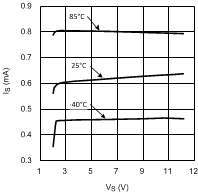Figure 1. Supply Current vs Supply Voltage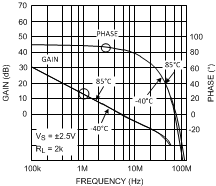Figure 3. Gain and Phase vs Frequency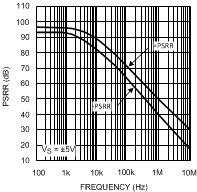Figure 5. PSRR vs Frequency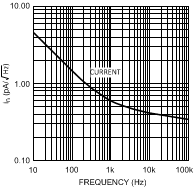Figure 7. Input Current Noise vs Frequency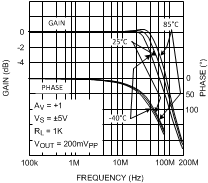Figure 9. Frequency Response For Various (AV)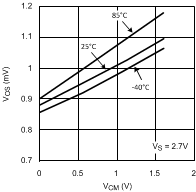Figure 11. Offset Voltage vs Common Mode Voltage
(a Typical Unit)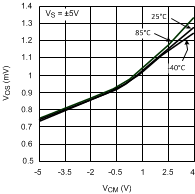Figure 13. Offset Voltage vs Common Mode Range
(a Typical Unit)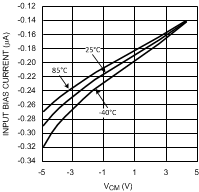Figure 15. Input Bias Current vs VCM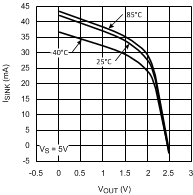Figure 17. Sink Current vs VOUT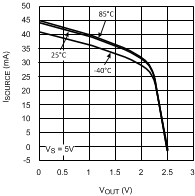Figure 19. Source Current vs VOUT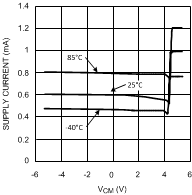Figure 2. Supply Current vs VCM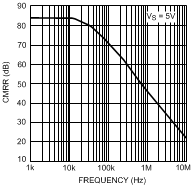Figure 4. CMRR vs FrequencyFigure 6. Input Voltage Noise vs Frequency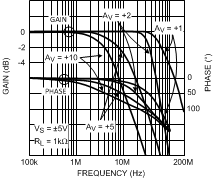Figure 8. Closed-Loop Frequency Response for Various Temperature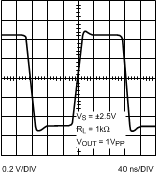Figure 10. Large Signal Step Response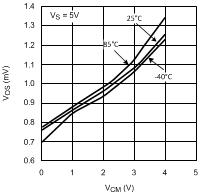Figure 12. Offset Voltage vs Common Mode Voltage (a Typical Unit)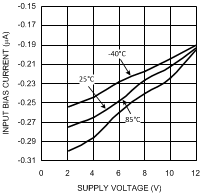Figure 14. Input Bias Current vs Supply Voltage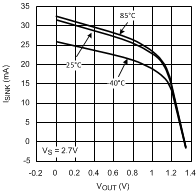Figure 16. Sink Current vs VOUT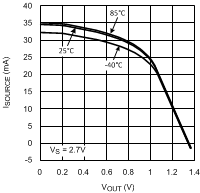Figure 18. Souce Current vs VOUT